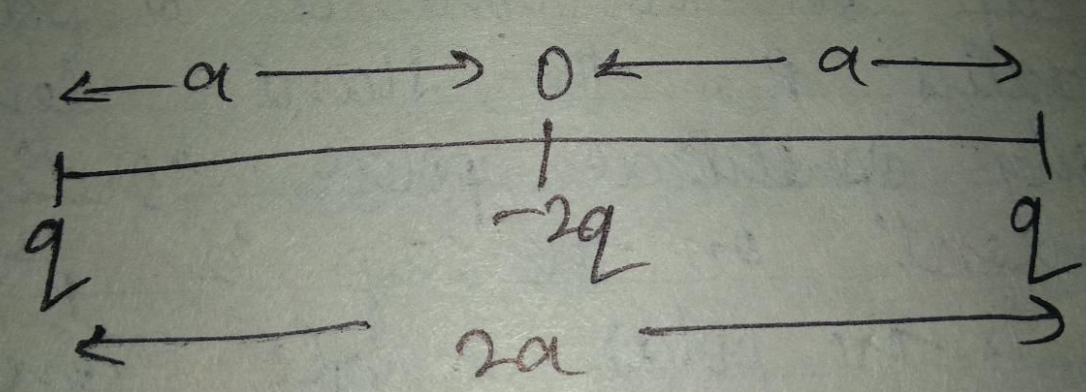Question

Two equal charges $$q$$ are placed at a distance $$2a$$ and a third charge $$-2q$$ is placed at the midpoint. The potential energy of the system is

A
9q28πϵ0aB
q28πϵ0aC
7q28πϵ0aD
6q28πϵ0aSolution

The correct option is C $$\dfrac{-7q^2}{8\pi\epsilon_0a}$$Given two equal charges $$'q'$$ are at a distance $$2a$$.A third charge $$-2q$$ is placed at the mid-point. We have to find the potential energy of the system.Let $$O$$ be the midpoint of the charges.So, potential energy of the system is$$=\dfrac{1}{4\pi \varepsilon _{0}}\left [ \dfrac{(-2q)(q)}{a}-\dfrac{(2q)(q)}{a}+\dfrac{(q)(q)}{2a} \right ]$$$$=\dfrac{1}{4\pi \varepsilon _{0}}\left [ \dfrac{-2q^{2}}{a}-\dfrac{2q^{2}}{a}+\dfrac{q^{2}}{2a} \right ]$$$$=\dfrac{1}{4\pi \varepsilon _{0}}\left [ \dfrac{-4q^{2}}{a}+\dfrac{q^{2}}{2a} \right ]$$$$=\dfrac{1}{4\pi \varepsilon _{0}}\left [ \dfrac{-8q^{2}a+q^{2}a}{2a^{2}} \right ]$$$$=\dfrac{1}{4\pi \varepsilon _{0}}\left [ \dfrac{-7q^{2}a}{2a^{2}} \right ]$$$$=\dfrac{1}{4\pi \varepsilon _{0}}\left [ \dfrac{-7q^{2}}{2a} \right ]$$$$=\dfrac{-7q^{2}}{8\pi \varepsilon _{0}a}$$Physics

Suggest Corrections0Similar questions
View MorePeople also searched for
View More